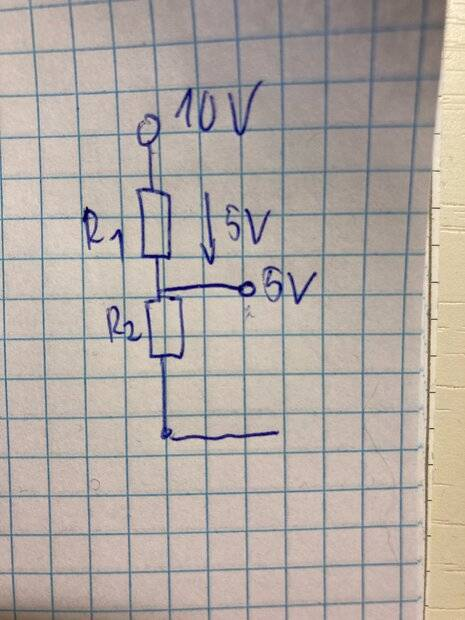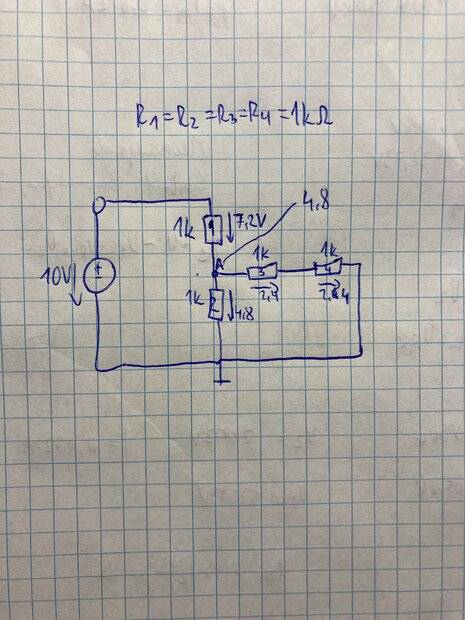# Simple voltage divider -- why does this work?

• altruan23
This becomes: ##V_2 = V_0 \times \frac {R_A}{R_1 + R_A}= 12 \times \frac {1}{1 + \frac {2k||1k}} = 6.7V##In summary, the voltage divider will work correctly only if the currents through the resistors are equal. If the currents through the resistors are not equal, then the voltage divider will not work correctly and you will need to use another way to generate the 5V.f

#### altruan23

Summary:: don't understand how can we use a voltage that has been already dropped.I don't understand the voltage divider. So R1 and R2 are the same. I have 10 V in beginning, then 5V drop across R1 and then i have 5V drop across R2. But how can I use the 5V if they already dropped at R2? If i connect to the 5V node another resistor in series for example, will 5V drop again across R2? How can we use the 5V, if they drop across R2 always??

You understand correctly that hooking anything up to the 5V point will have an effect on the circuit, but it depends on what that circuit is as to whether or not the voltage divider is a bad idea. If, for example, you were just forward biasing the base of an NPN transistor through a high resistance it would be fine. If on the other hand you were trying to power a significant 5V circuit, it would not work well at all.

Last edited:
•FactChecker, Joshy and altruan23So i start with 10V, i get a voltage drop on R1 --> 7.2V
Remainder is 4.8V which drops on R2. How do we have in node A 4.8V and then they drop across R3 and R4, if the 4.8V already dropped across R2 in the first loop??

Your analysis is incorrect. The "drop" across R3 and R4 has to be the same as across R2 because the total around any closed loop has to be 0. You need to show your method in detail. Kirchhoff.

•altruan23
First, there's an error in your analysis since ##4.8+7.2 \neq 10V##. The voltage drops must be equal, or, as KVL says, the voltages around any loop in the circuit must sum to zero. Try again.

The voltage at node ##A## is indeed the voltage across both ##R2## and across ##R3+R4##. The voltage isn't lost when it's "dropped", that is just means "voltage difference across components".

•altruan23
Summary:: don't understand how can we use a voltage that has been already dropped.

View attachment 297782
I don't understand the voltage divider. So R1 and R2 are the same. I have 10 V in beginning, then 5V drop across R1 and then i have 5V drop across R2. But how can I use the 5V if they already dropped at R2? If i connect to the 5V node another resistor in series for example, will 5V drop again across R2? How can we use the 5V, if they drop across R2 always??

I think your intuition is correct here. You will only have 5V at that central node if there are no other components connected to that node.

You can still "use" the voltage at that node if, for example, you add another resistor in parallel with R2, but that will cause the voltage to decrease at that node. This is because some of the current that was originally flowing through R2 to generate the 5V across it will now be diverted to flow through the new load. There will still be some useable voltage there, but it will be decreased in proportion to the amount of current that your additional load requires. Then the voltage across R1 will increase because of that decreased voltage.

Last edited:
•altruan23
sorry i wanted to write 12V instead of 10V

How useful and stable the 5 volt supply depends on the resistance of the circuit connected to it. You can count on 5 volts if the connected circuit resistance is high enough so that the voltage drop is not significant.

Can I add a few words.

Assume the supply is 12V.

The simple voltage divider formula for the Post #1 circuit gives:
##V_2 = V_0 \times \frac {R_2}{R_1 + R_2}= 12 \times \frac {1}{1 + 1} = 6V##

But the voltage divider formula only works correctly when there are equal currents through the series resistors. (Nearly equal currents will give an approximate answer which may be accurate enough in many situations.)

For the Post#1circuit, the same current flows through ##R_1## and ##R_2## so there is no problem

But for the Post #3 circuit, the current through ##R_2## is less than the current through ##R_1##. So we can’t use the voltage divider formula unless we allow for the additional resistors.

In the Post #3 circuit, the bottom three resistors have a total resistance (say ##R_A##) ##= (2k||1k) = \frac 2 3 k##.

So to use the voltage divider formula correctly for the Post #3 circuit, you must treat R_1 and R_A as the two series resistors.

•altruan23 and DaveE